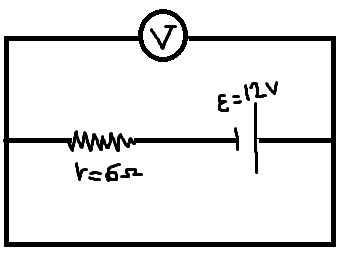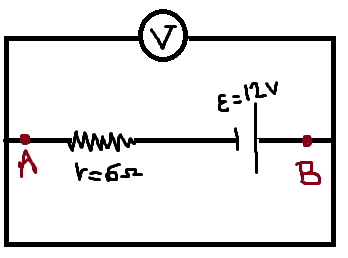# Current in a circuit without external resistance

• MatinSAR
In summary, the conversation discusses the concept of current in a circuit where the potential difference between its two ends is 0. The speaker explains that the equation i=ε/r does not predict a specific value for the current in this situation, and that the shape of the circuit in a schematic is irrelevant. They also mention the possibility of superconductors, which can maintain a current with no applied voltage. The conversation ends with a brief discussion of Kirchhoff's Voltage Law and the concept of equilibrium current.f

#### MatinSAR

Homework Statement
What number does the voltmeter show?
Relevant Equations
i=ε/rIt shows 0 and it's obvious why. I have another question.
Can I say that in this circuit the current is maximum and it equals to ##\frac ε r = 2A##?!Are A and B two ends of the wire? If yes, Why is there current in the wire even though the potential difference between its two ends is 0?
I think this ambiguity is created because the shape of the given circuit is different from the real circuit. In reality, the internal resistance is not visible and the two ends of the wire are connected to the positive and negative terminals, so the potential difference is not 0. Am I right?

Why is there current in the wire even though the potential difference between its two ends is 0?
Because when a is connected to b initially there is a 12 volt drop across the resistor which drops to zero at a rate of 63% every R⋅C seconds. Where C is the capacitance and R is the resistance.

Edit: Oh my. I took the battery as a capacitor. My response should have been: Because when a is connected to b initially there is a 12 volt drop across the resistor.

Last edited:
•DaveE and MatinSAR
and the two ends of the wire are connected to the positive and negative terminals
It's a circuit diagram; there may not even be a wire in a realization of this circuit: if the terminals of the resistor are connected directly to the terminals of the battery, the circuit is still represented by the same diagram, and points A and B would be one and the same point !

##\ ##

•MatinSAR
Homework Statement: What number does the voltmeter show?
Relevant Equations: i=ε/r

Why is there current in the wire even though the potential difference between its two ends is 0?
The equation:$$E=IR$$makes no prediction for ##I## when both ##E## and ##R## are zero. The equation is consistent with any current.

The shape of the circuit presented in a schematic is irrelevant. However, human intuition is easily confused by the layout choices on a schematic.

But we've not confused you -- you've correctly calculated how much current will flow in this particular bare wire.

An easier example with no chance for confusion is a simple loop of wire. Any current at all could be flowing around the loop. This is physically possible.

https://en.wikipedia.org/wiki/Superconductivity#Zero_electrical_DC_resistance said:
Superconductors are also able to maintain a current with no applied voltage whatsoever, a property exploited in superconducting electromagnets such as those found in MRI machines. Experiments have demonstrated that currents in superconducting coils can persist for years without any measurable degradation. Experimental evidence points to a current lifetime of at least 100,000 years. Theoretical estimates for the lifetime of a persistent current can exceed the estimated lifetime of the universe, depending on the wire geometry and the temperature. In practice, currents injected in superconducting coils have persisted for more than 27 years (as of August, 2022) in superconducting gravimeters. In such instruments, the measurement principle is based on the monitoring of the levitation of a superconducting niobium sphere with a mass of 4 grams.
Here we see estimates with varying degrees of reliability. 27 years for actual observation. 100,000 years based on extrapolation from experiment and "lifetime of the universe" based on theoretical expectation.

As I understand it, what you do in practice is to start with a warm loop in a strong magnetic field. Then you chill the loop while maintaining the magnetic field. Now you have a superconducting loop carrying zero current. You extinguish the magnetic field. The change in magnetic flux excites a current. Now you have a superconducting loop carrying non-zero current.

Last edited:
•MatinSAR
•BvU
OK, let's try an explanation that doesn't include things like magnetic fields and capacitance, which you didn't ask about....

Your result is the necessary conclusion of Kirchhoff's Voltage Law.

First of all, except for superconductors, there is always some resistance in any connection. In schematics it is understood that it is so small we don't have to show it; i.e. small compared to 6Ω, in your case. Let's say it's 1mΩ, then the current would be actually 12V/6.001Ω = 1.9997A. It is unusual for engineers to care about such a small difference, but if they did, they would need to show that 1mΩ in their schematic.

The superconducting case (0Ω) is a bit more odd but it still works. Suppose there was 2A flowing and for some reason it "decided" to decrease to 1.99A. Then the voltage across the short circuit would rise to 12V - 1.99A⋅6Ω = 0.06V. Then the resulting additional 0.06 volts across the connection will make the current increase back to the stable value of 2A. The same thing happens with a momentary increase in the current. There is an equilibrium value of current and any deviation from that will result in a voltage to restore the balance.

So, there is only one solution that creates the voltage balance that Kirchhoff described. For a superconductor it's 2A with 0V. For the 1mΩ "short circuit" that balance happens with 1.9997A and 1.9997mV.

Last edited:
••BvU, jbriggs444 and MatinSAR
••